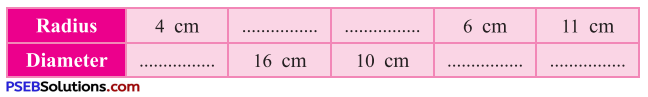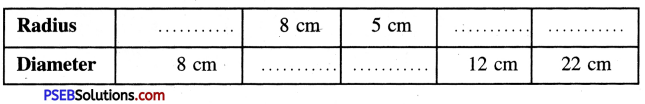# PSEB 4th Class Maths Solutions Chapter 7 Shapes Ex 7.1

Punjab State Board PSEB 4th Class Maths Book Solutions Chapter 7 Shapes Ex 7.1 Textbook Exercise Questions and Answers.

## PSEB Solutions for Class 4 Maths Chapter 7 Shapes Ex 7.1

Question 1.
From the given figure write the names of the following :
(b) diameter
(c) chords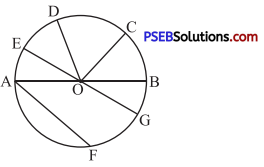Solution:
(a) Radius = OC, OB, OG, OD, OE, OA
(b) Diameter = AB,EG .
(c) Chords = AF, AB, EG.

Question 2.
Find the radius of a circle whose diameter is :
(a) 6 cm
(b) 8.2 cm
(c) 8.6 cm
Solution:
(a) Diameter of the circle = 6 cm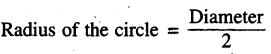= $$\frac{6}{2}$$ cm = 3 cm

(b) Diameter of the circle = 8.2 cm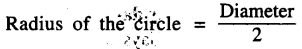= $$\frac{8.2}{2}$$ cm = 4.1 cm

(c) Diameter of the circle = 8.6 cm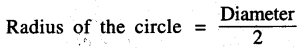= $$\frac{8.6}{2}$$ cm = 4.3 cmQuestion 3.
Find the diameter of a circle whose radius is :
(a) 13 cm
(b) 21 cm
(c) 17 cm
(d) 8 cm
Solution:
(a) Radius of the circle = 13 cm
Diameter of the circle = 2 × Radius
= 2 × 13 cm = 26 cm
(b) Radius of the circle = 21 cm
Diameter of the circle = 2 × Radius
= 2 × 21 cm
= 42 cm
(c) Radius of thte circle = 17 cm
Diameter of the circle = 2 × Radius
= 2 × 17 cm
= 34 cm
(d) Radius of the circle = 8 cm
Diameter of the circle = 2 × Radius
= 2 × 8 cm
= 16 cm

Question 4.
With the help of a compass draw a circle whose radius is :
(a) 5 cm
(b) 3 cm
(c) 2 cm
(d) 3.5 cm
(e) 4.6 cm
(f) 2.5 cm
Solution: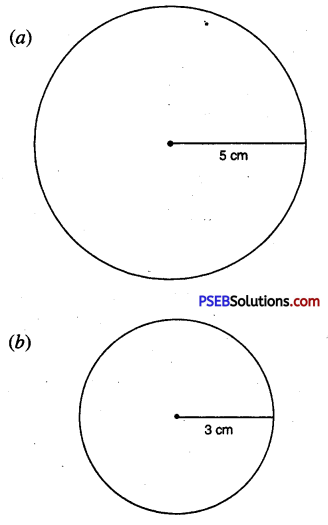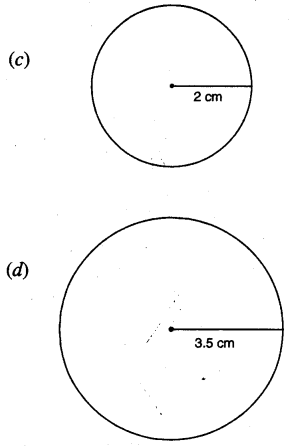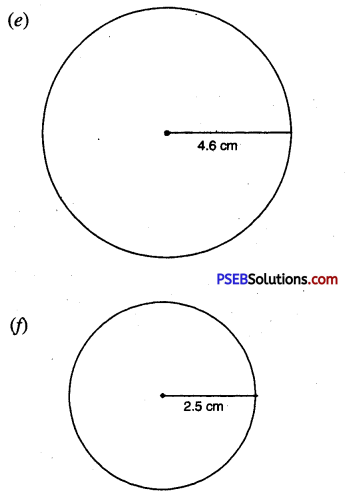Question 5.
Which is the longest chord of a circle ?
Solution:
Diameter.Question 6.
Fill in the blanks :
(a) A line segment which joins centre of a circle with any point on circumference is called …………
(b) Diameter of a circle = ………. × radius.
(c) The longest chord of a circle is called …….. of circle.
(d) All the radii of circle are …… in length.
Solution: